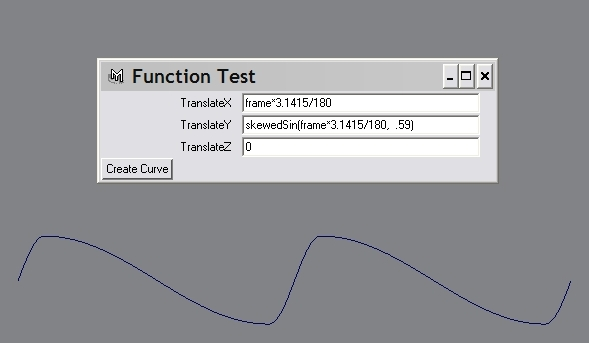Class 2
Let us continue writing more small programs to get familiar with the syntax and more importantly, with MEL function calls ("commands") that are built-in to enable you to do a variety of scene-related tasks.
1. Write an 'autoUI_2.mel' program to create a UI for keyable attrs of a selected list of objects. Note - this is a slightly different program compared to the one from Class 1. The UI now should look like so: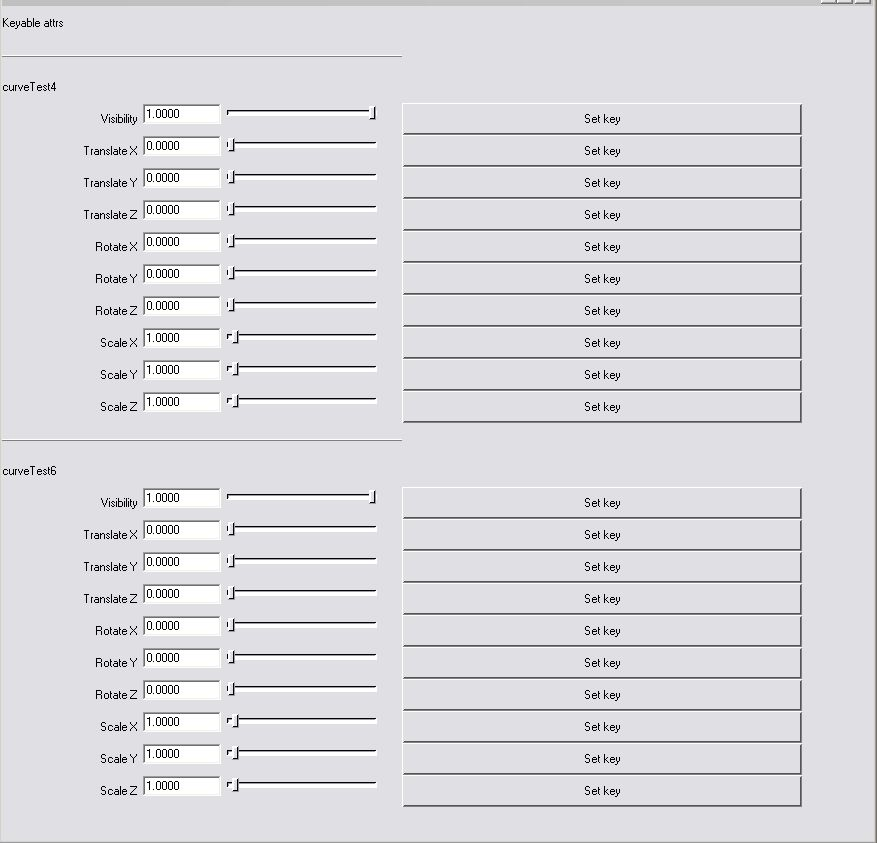Usage: autoUI_2

Useful commands:

• window
• gridLayout
• text
• button
• separator
• attrFieldSliderGrp
• showWindow
• listAttr
• setKeyframe
2. Write a 'roughenSurf.mel' program to add noise to CVs of a NURBS surface, thereby creating some modelled texture. Such "script deformers" are really useful to learn to write - you can create waves, facial animation, surface patterns, cloth-like animation, etc. simply by displacing CVs yourself (these would serve as a prototype for plugins which would do the same things faster).

Usage: roughenSurf()

Useful commands:

• getAttr
• xform
• noise
Exercise:
Apply 3D vector noise (solid noise) to vertices of a polymesh head, to generate variations like these: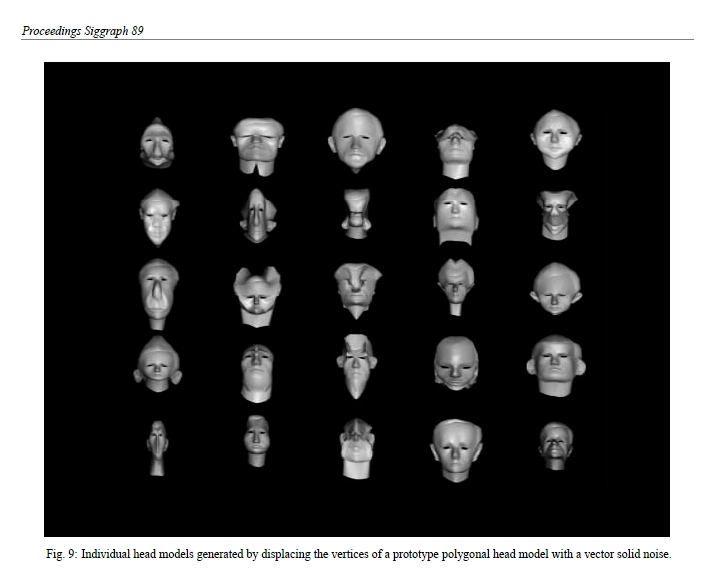The above image is from John Lewis' 1989 SIGGRAPH paper 'Algorithms for Solid Noise Synthesis'.

3. Write an 'plotFunc.mel' program to let you experiment with plotting 2D and 3D curves. Needless to say, this is REALLY useful for animating practically every attribute in every node! The UI should look like this: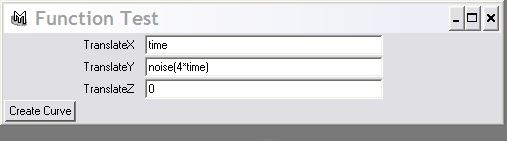Usage: plotFunc()

Useful commands:

• window
• showWindow
• columnLayout
• textFieldGrp
• button
• curve
• expression
• playbackOptions

```// Here's how to plot a square wave, using Fourier synthesis.
// Sine waves of increasing freq. and decreasing amplitudes are
// summed to approximate an ideal square wave.
// Declare sqWave [code below], then do (with 360 frames):
// X: frame
// Y: sqWave(time,2); // try 0,1,2,3.. for the 2nd argument
// Z: 0
proc float sqWave(float \$t, int \$n)
{
float \$val=0.0;

for(\$i=0;\$i<=\$n;\$i++)
{
int \$m = (1+2*\$i);
print(\$m + "\n");
\$val += 4/3.14159278*(1.0/\$m*sin(\$m*\$t));
}
return \$val;

}// sqWave
```
Here's the result of varying \$n (summation count) to be 0,1,2,3,4,6,8,10,20,30,40,50.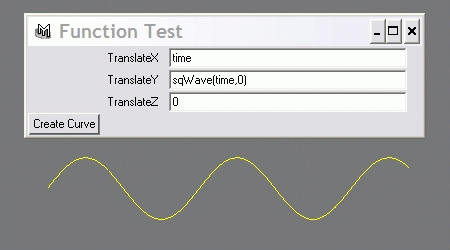Try the following (make sure your playback range is 1..1440 frames):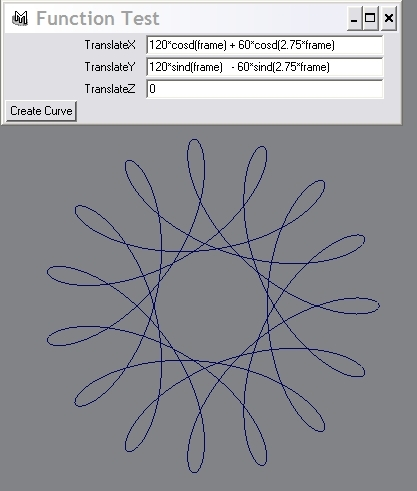4. Create a 'biasGain.mel' program that has a bias() and a gain() function. These two functions are great for 'nonlinearly' warping any quantity, eg. to produce ease-in/ease-out, gamma enhancement, etc.

Usage:
bias(inputVal,biasAmt);
gain(inputVal,gainAmt);

Useful commands:

• pow
• log
It might be also helpful to create a normTime() call to take the current frame, start frame and end frame values and use them to express current time as a 0..1 value.

Use the plotFunc plotter to visualize what bias() and gain() do..

5. Write a 'skewedSin.mel' program that creates a custom sin() curve where the 'peak' of the curve in the 0..180 deg. range does not necessarily occur at 90 degrees. In other words, the peak should be 'skewable' ( towards 0 deg. or 180 deg.) by the user.

Usage: skewedSin(myAngle, myPeak); //note: myAngle and myPeak should be radians (not degrees)

Useful commands:

• sin
Experiment by using skewedSin() instead of sin() in simple expressions that drive joint angles, camera rotation, etc. Also, visualize skewedSin() using your function plotter.

Here is a sample curve from skewedSin() [frame range is -360 to 360].